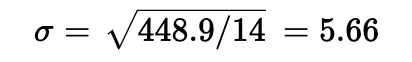top of page
Search

# How to Calculate Standard Deviation with Formulas

Updated: Dec 21, 2022

### Introduction to Standard Deviation and When It's Used

Standard deviation is a statistical measure that tells you how spread out the data points are around the mean. The higher the standard deviation, the more spread out the data points are.

The most common use of standard deviation is to determine if a set of scores is normally distributed. If you have a set of scores that have a high degree of variability, it may be difficult to tell if they are normally distributed or not. A high degree of variability usually indicates that there's something different about those scores, such as outliers or skewness.

Standard deviation is a statistical measure that is used to describe the amount of variation or dispersion in a set of data. It is calculated by finding the difference between each data point and the mean (average) of the data set, and then finding the square of those differences. The resulting values are then summed and divided by the number of data points, and the square root of that value is the standard deviation.

Standard deviation is a useful measure because it gives a sense of how much the individual data points in a set vary from the mean. A small standard deviation indicates that the data points are close to the mean, while a large standard deviation indicates that the data points are more spread out. Standard deviation is often used in statistical analysis to understand the distribution of data and to make predictions about future data points. It is also used to assess the reliability of statistical conclusions that are based on a sample of data.

 Sr No Height (x) x - x̅ (x-x̅)² 1 160 -8.29 68.7 2 170 ​1.71 2.9 3 167 ​-1.29 1.7 4 164 ​-4.29 18.4 5 172 ​3.71 13.8 6 174 5.71 32.7 7 169 0.71 0.5 8 173 4.71 22.2 9 175 6.71 45.1 10 166 -2.29 5.2 11 158 ​-10.29 105.8 12 177 8.71 75.9 13 161 -7.29 53.1 14 170 1.71 2.9 ​ ​ ​ ​ N = 14 (Total Count) x̅ = 168.29 (mean/average) ​ ​∑(x-x̅)²=448.9### What is a Normal Distribution?

A normal distribution is a type of distribution that is bell shaped. This means that the data has a symmetrical and peaked shape. The mean, median, and mode are all equal to each other.

### How Does Standard Deviation Work? What Makes it Different from Mean or Variance?

Standard deviation is a statistical measure that tells us how much the data in a set of numbers deviate from the average.

It is different from mean and variance because it measures how dispersed the data in a set of numbers is around an average, rather than just telling us what that average is.